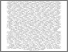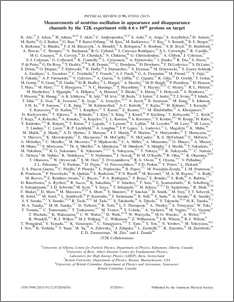# Measurements of neutrino oscillation in appearance and disappearance channels by the T2K experiment with 6.6E20 protons on target

, T2K Collaboration (2015) Measurements of neutrino oscillation in appearance and disappearance channels by the T2K experiment with 6.6E20 protons on target. Physical Review D, 91 (7). ISSN 1550-7998Preview
PDF (PhysRevD.91.072010)
PhysRevD.91.072010.pdf - Published Version

## Abstract

We report on measurements of neutrino oscillation using data from the T2K long-baseline neutrino experiment collected between 2010 and 2013. In an analysis of muon neutrino disappearance alone, we find the following estimates and 68% confidence intervals for the two possible mass hierarchies: Normal Hierarchy: $\sin^2\theta_{23}=0.514^{+0.055}_{-0.056}$ and $\Delta m^2_{32}=(2.51\pm0.10)\times 10^{-3}$ eV$^2$/c$^4$ Inverted Hierarchy: $\sin^2\theta_{23}=0.511\pm0.055$ and $\Delta m^2_{13}=(2.48\pm0.10)\times 10^{-3}$ eV$^2$/c$^4$ The analysis accounts for multi-nucleon mechanisms in neutrino interactions which were found to introduce negligible bias. We describe our first analyses that combine measurements of muon neutrino disappearance and electron neutrino appearance to estimate four oscillation parameters and the mass hierarchy. Frequentist and Bayesian intervals are presented for combinations of these parameters, with and without including recent reactor measurements. At 90% confidence level and including reactor measurements, we exclude the region: $\delta_{CP}=[0.15,0.83]\pi$ for normal hierarchy and $\delta_{CP}=[-0.08,1.09]\pi$ for inverted hierarchy. The T2K and reactor data weakly favor the normal hierarchy with a Bayes Factor of 2.2. The most probable values and 68% 1D credible intervals for the other oscillation parameters, when reactor data are included, are: $\sin^2\theta_{23}=0.528^{+0.055}_{-0.038}$ and $|\Delta m^2_{32}|=(2.51\pm0.11)\times 10^{-3}$ eV$^2$/c$^4$.

Item Type:
Journal Article
Journal or Publication Title:
Physical Review D
©2015 American Physical Society 111 pages, 45 figures, submitted to Physical Review D. Minor revisions to text following referee comments
Subjects:
Departments:
ID Code:
73766
Deposited By:
Deposited On:
18 Jun 2015 05:44
Refereed?:
Yes
Published?:
Published